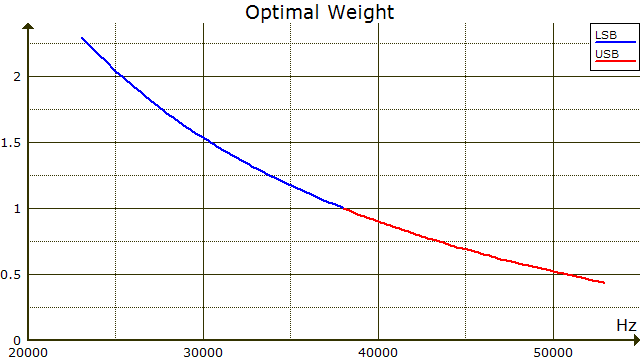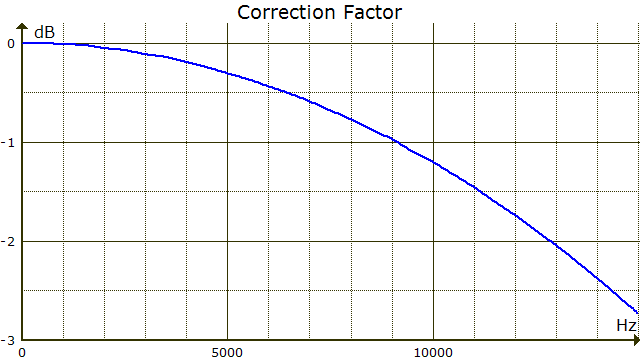# Alternative Stereo Decoding Methods

Occasionally it's suggested that FM stereo noise could be reduced by decoding only the lower sideband of the double-sideband L−R signal. Since this requires half the bandwidth, the noise should be half as much. In fact, goes the argument, detected FM noise actually rises with frequency, so USB noise is greater than LSB noise, yielding even further noise reduction.

What the argument neglects is that dispensing with the upper sideband forgoes the signal components there. During synchronous demodulation, LSB and USB signal components combine coherently, their amplitudes adding to yield 6 dB of gain. But uncorrelated noise in the two sidebands combines incoherently, increasing only 3 dB for flat noise. Still, FM noise does rise with frequency, so perhaps LSB decoding offers a benefit despite the 6-dB signal penalty.

I wrote a short computer program to resolve the issue. The program calculates S/N gain for three alternative stereo decoding methods. The first is LSB decoding. The second is optimal linear decoding, where the upper and lower sidebands are combined with weighting coefficients that maximize S/N for the combination. The coefficients vary with frequency so this method essentially multiplies the sidebands by a weighting curve before combining them.For two noisy channels with equal signal amplitudes, the optimal weighting coefficient for the quieter channel is n/q, where n and q are the RMS noise amplitudes of the noisier and quieter channels. The coefficient for the noisier channel is q/n. These coefficients don't sum to a constant value, so the signal spectrum is corrected to flat after combination.The third alternative method uses LSB decoding, but the transmit signal has twice-normal LSB amplitude and no USB. A multipath simulation for this signal is here.

Here are the results:

```Method                       S/N Gain
LSB decoding                 -2.28 dB
Optimal linear decoding       0.20
LSB decoding + LSB transmit   3.69
```

It's remarkable that the optimal linear decoder offers so little improvement over the standard decoder. The Pioneer F-93 tuner does LSB decoding, but for interference reduction, not S/N enhancement.

Since detected FM noise rises 6 dB per octave, the program uses frequency as noise amplitude. It integrates the noise powers, weighting them as required.

```	CONST fc = 1 / (2 * 3.141593 * 75E-6)	' Deemphasis corner frequency
DEF FNdb (p) = 10 * LOG(p) / LOG(10)	' Power -> dB, LOG is natural log

FOR f = 20 TO 15000			' Integrate noise components from 20 Hz to 15 kHz
d = 1 / (1 + f / fc * f / fc)		' Deemphasis filter power factor
b = b + d * f * f			' Accumulate L+R noise power
l = 38000 - f                         ' L-R LSB freq
u = 38000 + f                         ' L-R USB freq
nl = nl + d * l * l                   ' Accumulate deemphasized LSB noise power
nu = nu + d * u * u			' Accumulate deemphasized USB noise power
w = u / l                             ' Optimal weighting coefficient
l = l * w                             ' Weighted LSB noise amp
u = u / w                             ' Weighted USB noise amp
s = w + 1 / w                         ' Weighted signal amp (1 + 1 unweighted)
c = 2 / s                             ' Amplitude-correction factor for flat signal
nw = nw + d * (l * l + u * u) * c * c ' Weighted, corrected, deemphasized noise power
NEXT f

PRINT fndb((b + nl + nu) / (b + 4 * nl))' S/N gain for LSB decoding
PRINT fndb((b + nl + nu) / (b + nw))	' S/N gain for optimal linear decoding
PRINT fndb((b + nl + nu) / (b + nl))	' S/N gain for LSB decoding + LSB transmit
```

February 11, 201688–108 MHz